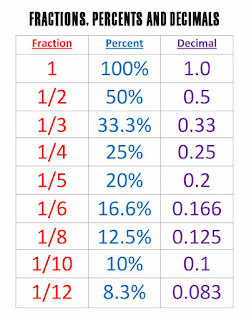# Why percentages are so confusing and how to make them painless

If you hate percentages and can’t seem to get your head around all of the different types of percentage questions, you’re not alone.

It can be a really confusing topic.

You might be tempted to avoid it and I don’t blame you at all.

Sadly, you won’t get far very far with GCSE Maths if you don’t learn how to do these questions.

I’ll do my best to simplify this topic and hopefully make it easier for you.

Let’s start by looking at what a percentage actually is.

The word percent means ‘Out of 100’. Per as in for each one. And cent, the french for 100.

The purpose of working out percentages is to help compare numbers and to make things simpler.

For example, when you go shopping, a sale sign that says 50% off is much clearer than a sign that says £37 off.

Percentages help us understand numbers faster.

Usually when you get a test result, you will have your score as well as a percentage.

Before you start working on how to calculate percentages, I highly recommend starting by learning this comparison table for fractions, percentages and decimals:Knowing the fraction equivalent of a percentage will help you when doing calculations.

How to calculate one number as a percentage of another:

When you get a test result at school or from your learning journey, you receive 2 numbers.

1. Your Score, the number of questions you got correct.

2. The total possible score or marks that you could have got.

Let’s say you do a test where the total possible marks are 100.

And you score 50 marks. This means you got 50 out of 100, or 50/100.

In other words you got half correct, which is 50%.

If your score was 25, this would be 25/100 which is 25%.

If your score was 54, this would be 54/100, which is 54%

If your score was 100, this would be 100/100, which is 100%.

Do you notice the pattern?

Anything out of 100, is already a percentage, because percentages are out of 100.

So 63/100, is 63%. 81/100 is 81% and so on.

Of course in the real world, most situations are not ‘out of 100’, and that’s where things start to get tricky.

In order to find a percentage, you need to multiply the numbers so that they are out of 100.

E.g what is 5 out 25 as a percentage?

Let’s write it as a fraction: 5/25.

Hopefully, you will notice that 25 goes very nicely into 100 4 times. So if we multiply both top and bottom numbers by 4, we will get the equivalent fraction of 20/100.

25/100 is 20%. This means that 5/25 is also 20%.

I hope that helps.

Below are some practice questions, in part 2 I will talk you through the percentage questions that don’t go so nicely into 100.

Change the following into percentages:

1. 4/20

2. 25/50

3. 3/4

4. 4/5

5. 37/50

6. 8/20

7. 24/25

8. 17/20

9. 8/10

10. 17/50

## One response

1. […] please see my previous posts on calculating percentages if you are unsure […]